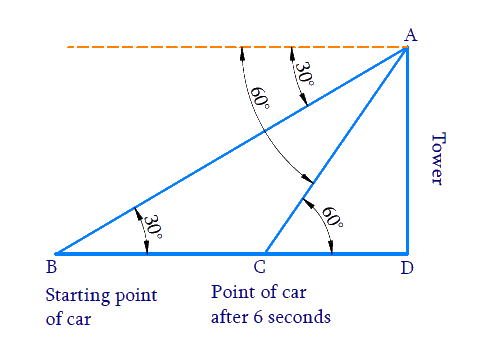# Ex.9.1 Q15 Some Applications of Trigonometry Solution - NCERT Maths Class 10

Go back to  'Ex.9.1'

## Question

A straight highway leads to the foot of a tower. A man standing at the top of the tower observes a car at an angle of depression of $$30^\circ$$, which is approaching the foot of the tower with a uniform speed. Six seconds later, the angle of depression of the car is found to be $$60^\circ.$$ Find the time taken by the car to reach the foot of the tower from this point.

Video Solution
Some Applications Of Trigonometry
Ex 9.1 | Question 15

## Text Solution

What is Known?

(i) Angle of depression is $$30^\circ$$

(ii) $$6$$ seconds later angle of depression is $$60^\circ$$

What is Unknown?

Time taken by the car to reach the foot of the tower $$= CD$$Reasoning:

Let the height of the tower as $$AD$$ and the starting point of the car as $$B$$ and after $$6$$ seconds point of the car as $$C$$. The angles of depression of the car from the top $$A$$ of the tower at point $$B$$ and $$C$$ are $$30^\circ$$ and $$60^\circ$$ respectively.

Distance travelled by the car from the starting point towards the tower in $$6$$ seconds $$= BC$$

Distance travelled by the car after 6 seconds towards the tower $$= CD$$

Trigonometric ratio involving $$AD, \,BC, \,CD$$ and angles is $$tan\,\theta$$.

We know that,

$\text {Speed} = \frac { \text { Distance } } { \text { Time } }$

The speed of the car is calculated using the distance $$BC$$ and time $$= 6\,\rm seconds.$$ seconds. Using Speed and Distance $$CD,$$ time to reach foot can be calculated.

Steps:

In $$\Delta ABD$$,

\begin{align}\tan {{30}^{0}}&=\frac{AD}{BD} \\ \frac{1}{\sqrt{3}}&=\frac{AD}{BD} \\BD&=AD\sqrt{3}\,\,\,....(1) \end{align}

In $$\Delta ACD$$,

\begin{align} \tan {{60}^{0}}&=\frac{AD}{CD} \\ \sqrt{3}&=\frac{AD}{CD} \\ AD&=CD\sqrt{3}\,\,\,....(2)\text{ } \end{align}

From equation ($$1$$) and ($$2$$)

\begin{align}BD&=CD\sqrt{3}\times \sqrt{3} \\ BC+CD&=3CD\\&\left[ \because BD=BC+CD \right] \\ BC&=2CD\,\,\,\dots(3)\end{align}

Distance travelled by the car from the starting point towards the tower in $$6$$ seconds $$= BC$$ Speed of the car to cover distance $$BC$$ in $$6$$ seconds;

\begin{align}\text Speed&=\,\frac{\text{Distance}}{\text{Time}} \\ & =\,\frac{BC}{6\,} \\ &=\,\frac{2CD}{6}\,\qquad\left[ \text{from(3)} \right] \\ & =\,\frac{CD}{3}\, \end{align}

Speed of the car =\,\begin{align}\frac{CD}{3}\,\rm{m/s}\end{align}

Distance travelled by the car from point $$C$$, towards the tower $$= CD$$

Time to cover distance $$CD$$ at the speed of =\,\begin{align}\frac{CD}{3}\,\rm{m/s}\end{align}

\begin{align}\text Time &=\frac{\text{Distance}}{\text{Speed}} \\ & =\frac{CD}{\frac{CD}{3}}\, \\ & =CD\times \frac{3}{CD} \\ & =3 \end{align}

Time taken by the car to reach the foot of the tower from point $$C$$ is $$3$$ seconds.

Learn from the best math teachers and top your exams

• Live one on one classroom and doubt clearing
• Practice worksheets in and after class for conceptual clarity
• Personalized curriculum to keep up with school